# On A Dynamic Model for Protein Synthesis

## Introduction

### Model Description:Classical TASEP Model

The process of translation can be divided into three main stages: initiation, elongation and termination. Our model mainly focuses on the description of the elongation process. In fact, during the elongation phase, ribosome moves along mRNA, and simultaneously adding amino acids to the chain of protein being synthesized.

In our modeling, the mRNA is viewed as a one-dimensional lattice and each codon is considered as a grid in the lattice, while a ribosome can be either viewed as an 'extended solid particle' with length l, i.e. it covers l sites in the strand of mRNA, or as a machine with intrinsic structure, which will be talked about later.

The classical model views each ribosome as an extended solid particle with length 1, and 12 is a frequently used value for l owing to observations from experiments. The position of a ribosome is represented by the index of the site covered by the left edge of the ribosome. When the ribosome rests at site i, it waits until an aa-bounded tRNA bounds with the i+1-th site of mRNA. Therefore, each site i is endowed with a probability pa(i) such that in the time interval Δt, the probability of a correct aa-tRNA bounds to the i+1-th site is pa(i). The probability per second ωa(i) is useful in the numerical computations. It is defined as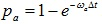. Thus the probabilities of hopping of a ribosome from i-th site to the next site is pa(i). An additional hypothesis is that the inter-ribosome interactions are neglected, except requiring that two different ribosomes cannot overlap. This makes the model easy to simulate. The system can be viewed as a TASEP(totally asymmetric exclusion process).

### Model Description: Mechano-Chemistry Details of Ribosome

However, some mechano-chemistry details for the motion of ribosomes on the mRNA strand should be taken into account.

We use the letters E, P and A to denote three binding sites on the ribosome. They look like sockets where the aa-tRNA's inserts into to bind with certain codons on mRNA. They can shift back and forth on mRNA and bring about the movement of ribosomes on the mRNA strand. In the figure, the states of E, P, A sites is presented by a number 0 or 1, representing the absence or presence of tRNA. 1* is a EF-Tu bound tRNA and G is a EF-G GTPase.

This gives an explicit description for these processes.

• Transition 1⇒2: Binding of a fresh tRNA-EF-Tu complex to site A;
• Transition 2⇒3: GTP part of EF-Tu hydrolized to GDP;
• Transition 3⇒4: Phosphate group, a product of the hydrolysis, leaves, and releases the EF-Tu;
• Transition 4⇒5: Shift of tRNA, and site A occupied by EF-G in the GTP bound form;
• Transition 5⇒6: Hydrolysis of GTP to GDP and release;
• Transition 6⇒7⇒1: Shift of ribosome.

### How to Interpret the Parameters?

We consider the system as a stochastic process, and the probability of a ribosome to change its state is described by the rate constants ω. Now we interpret these parameters:

For the simplest chemical reaction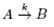with reaction rate k, by law of mass actions, we see that: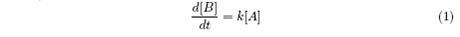Therefore, in time interval Δt, the probability of A to transform into B should be: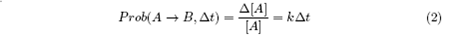Hence, if we set ω = k, ω can be interpreted as the probability "per Δt" for the reaction to occur on A. While the actual probability should be p = ωΔt or with more accuracy,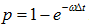.

The rate constants can be obtained from experiments for E-coli as: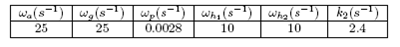Moreover, the initiation and termination process are described by two parameters α and β, denoting the reaction rate of a fresh ribosome binds to the first l sites of mRNA and the probability of a ribosome at the rear of the mRNA strand to get detached from the mRNA strand in the time interval Δt.

## Theoretical Analysis of the Model

We first specify some assumptions and notations. Pμ(i) with μ denotes the probability of finding a ribosome in state μ which is 'working' on site i and is assumed to be the site which is covered by the leftmost site of the ribosome.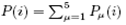is the probability of finding a ribosome at i. The ribosome can shift forward only if the sites with index from i+1 to i+l are all empty. Assume that the probabilities of finding a ribosome at any sites are independent. Then the probability Q(i) for the required sites are empty is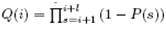.

Thus the differential equations are: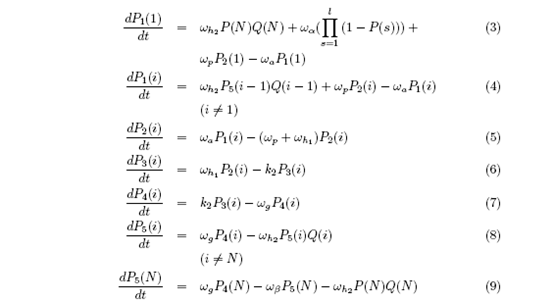Use Runge-Kutta method to get the solution. Here we assume that, if there were no detachments, the ribosomes are completely recycled.

However, this will not be used in our programming.

# Monte Carlo Methods

We use Monte Carlo method in our programming for convenience. Here is the specification of the algorithm:

Note that Each Monte Carlo Step(MCS) represents a time interval Δt = 0.001s in our simulation.

The mRNA strand of N codons is implemented by an array mRNA of length N, which takes value from 0 to 5 in each entry. 1 to 5 represents the state of ribosome on the certain position, while 0 represents the site is empty, or is not occupied by a "working" ribosome.

1. Set the initial condition: all the sites on the mRNA strand is empty except the site in the first entry.

2. Begin a new MCS:

a) Select an entry with value 1 (ribosome in state 1), generate a random number. If the random number is less than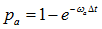, then change the value to 2, otherwise proceed;

b) Select an entry with value 2 (ribosome in state 2), generate a random number. If the random number is less than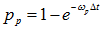, then change the value to 1, otherwise proceed;

c) Select an entry with value 2 (ribosome in state 2), generate a random number. If the random number is less than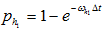, then change the value to 3, otherwise proceed;

d) Select an entry with value 3 (ribosome in state 3), generate a random number. If the random number is less than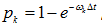, then change the value to 4, otherwise proceed;

e) Select an entry with value 4 (ribosome in state 4), generate a random number. If the random number is less than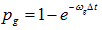, then change the value to 5, otherwise proceed;

f) Select an entry with value 5 (ribosome in state 5), generate a random number. If the random number is less than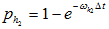, and that assume the index of the selected entry is i, if entries with index from i+1 to i+l are all of value 0, then change mRNA[i] to 0 and mRNA[i+1] to 1;

g) Look at the last entry, assume it has index N (when use MATLAB to program) or index N-1 (when use C++ to program), generate a random number. If the value of the last entry is 5 and the random number is less than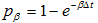, then change the last entry to 0.

h) Look at the first a few entries. If the first l entries are 0, then generate a random number. If the random number is less than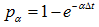, then change the first entry to 1.

3. Iterate Step 2, until maximal number of Monte Carlo Steps are reached.